Also found in: Dictionary, Thesaurus, Wikipedia.
Related to Planck's radiation law: photoelectric effect

A law of physics which gives the spectral energy distribution of the heat radiation emitted from a so-called blackbody at any temperature. Discovered by Max Planck, this law laid the foundation for the advent of the quantum theory because it was the first physical law to postulate that electromagnetic energy exists in discrete bundles, or quanta. See Heat radiation, Quantum mechanics

McGraw-Hill Concise Encyclopedia of Physics. © 2002 by The McGraw-Hill Companies, Inc.

See black body.
Collins Dictionary of Astronomy © Market House Books Ltd, 2006
The following article is from The Great Soviet Encyclopedia (1979). It might be outdated or ideologically biased.

the law of energy distribution in the spectrum of equilibrium radiation (electromagnetic radiation in thermodynamic equilibrium with matter) at a certain temperature. It was first derived by M. Planck in 1900 on the basis of the hypothesis of energy quanta. The law gives the spectral dependence of the energy density p (the energy of the radiation per unit volume) on the frequency v or wavelength λ = c/v, where c is the speed of light. Since the blackbody emissive power u = (c/4)ρ (the radiant energy emitted from the surface per unit area and time) is proportional to the energy density, it is also given by the law. The functions ρv, T and uv, T or ρλ, T and uλ, T for a unit interval of the frequencies or wavelengths are universal functions of v or X and of T; they are independent of the nature of the substance with which the radiation is in equilibrium.

Planck’s radiation law is expressed by the formula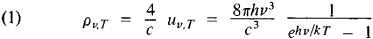or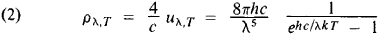where h is Planck’s constant and k is the Boltzmann constant. Function (2) is illustrated for various temperatures in Figure 1. The maximum of the function shifts toward the short-wavelength end of the spectrum with increasing T.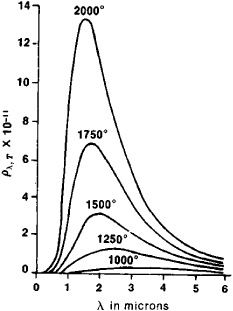Figure 1

Other laws of equilibrium radiation result from Planck’s radiation law. Integration with respect to v (or λ) from 0 to ∞ gives the values of the total energy density of radiation for all frequencies—the Stefan-Boltzmann radiation law: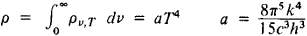We also obtain the total emissive power of a black body: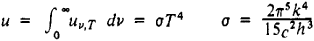In the high-frequency region the energy of the photon is much greater than the thermal energy (hv ≫ kT), and Planck’s radiation law becomes Wien’s radiation law:

ρv, T = (8πhv3/c3)e–hv/kT

In the low-frequency region, where kThv, it becomes the Rayleigh-Jeans radiation law:

ρv, T = (8πv2/c3)kT

These laws are thus the limiting cases of Planck’s radiation law. Wien’s displacement law also follows from Planck’s radiation law, which may be represented in the form pv, T = v3f(v/t), where f(v/T) is a function only of the ratio of v and T.

Planck’s radiation law is in agreement with experimental data and can be used to calculate the values of h and k. The temperatures of heated bodies—for example, the surfaces of stars—can be determined on the basis of the law by means of pyrometers. For temperatures greater than 2000°K, the only reliable method of temperature determination makes use of the blackbody radiation laws and Kirchhoffs radiation law. Planck’s radiation law is also used in calculations of light sources.

The law was derived by A. Einstein in 1916 through the examination of the quantum transitions for atoms in equilibrium with radiation. Planck’s radiation law can also be obtained as a consequence of the Bose-Einstein statistics.

M. A. EL’IASHEVICH